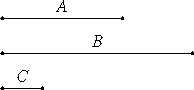# Proposition 29

Any prime number is relatively prime to any number which it does not measure.

Let A be a prime number, and let it not measure B.

I say that B and A are relatively prime.Def.VII.11

If B and A are not relatively prime, then some number C measures them.

Since C measures B, and A does not measure B, therefore C is not the same as A.

Now, since C measures B and A, therefore it also measures A which is prime, though it is not the same as it, which is impossible. Therefore no number measures B and A.

Def.VII.12

Therefore A and B are relatively prime.

Therefore, any prime number is relatively prime to any number which it does not measure.

Q.E.D.

## Guide

In effect, this proposition says that the only way a prime number can fail to be relatively prime to another number is if it divides that other number.

This proposition is used in the next one and in propositions IX.12 and IX.36.